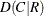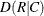# The FREQ Procedure

### TEST Statement

• TEST test-options;

The TEST statement requests asymptotic tests for measures of association and measures of agreement. The test-options identify which tests to compute. Table 40.20 lists the available test-options, together with their corresponding TABLES statement options. Descriptions of the test-options follow the table in alphabetical order.

For each measure of association or agreement that you request in the TEST statement, PROC FREQ provides an asymptotic test that the measure is 0. The procedure displays the asymptotic standard error under the null hypothesis, the test statistic, and the one-sided and two-sided p-values. PROC FREQ also provides confidence limits for the measure. The ALPHA= option in the TABLES statement determines the confidence level; by default, ALPHA=0.05, which provides 95% confidence limits. For more information, see the sections Asymptotic Tests and Confidence Limits. For information about the individual measures, see the sections Measures of Association and Tests and Measures of Agreement.

You can also request exact tests for selected measures of association and agreement by using the EXACT statement. For more information, see the section Exact Statistics.

#### Using the TEST Statement with the TABLES Statement

You must use a TABLES statement with the TEST statement. If you use only one TABLES statement, you do not need to specify the same options in both the TABLES and TEST statements; when you specify an option in the TEST statement, PROC FREQ automatically invokes the corresponding TABLES statement option. However, when you use the TEST statement with multiple TABLES statements, you must specify options in the TABLES statements to request statistics; PROC FREQ then provides asymptotic tests for those statistics that you specify in the TEST statement.

Table 40.20: TEST Statement Options

Test Option

Asymptotic Tests

Required TABLES Statement Option

Simple and weighted kappa coefficients

Gamma

Simple kappa coefficient

Kendall’s tau-b

Gamma, Kendall’s tau-b, Stuart’s tau-c,

Somers’, Somers’,

Pearson and Spearman correlations

Pearson correlation coefficient

Polychoric correlation

Spearman correlation coefficient

Somers’Somers’Stuart’s tau-c

Weighted kappa coefficient

You can specify the following test-options in the TEST statement.

AGREE

requests asymptotic tests for the simple kappa coefficient and the weighted kappa coefficient. For more information, see the sections Simple Kappa Coefficient and Weighted Kappa Coefficient.

The AGREE option in the TABLES statement provides estimates, standard errors, and confidence limits for kappa coefficients. You can request exact tests for kappa coefficients by using the EXACT statement.

Kappa coefficients are defined only for square tables, where the number of rows equals the number of columns. Kappa coefficients are not computed for tables that are not square. Fortables, the weighted kappa coefficient is identical to the simple kappa coefficient, and PROC FREQ presents only the simple kappa coefficient.

GAMMA

requests an asymptotic test for the gamma statistic. For more information, see the section Gamma. The MEASURES option in the TABLES statement provides the gamma statistic and its asymptotic standard error.

KAPPA

requests an asymptotic test for the simple kappa coefficient. For more information, see the section Simple Kappa Coefficient.

The AGREE option in the TABLES statement provides the kappa statistic, its standard error, and its confidence limits. You can request an exact test for the simple kappa coefficient by specifying the KAPPA option in the EXACT statement.

Kappa coefficients are defined only for square tables, where the number of rows equals the number of columns. PROC FREQ does not compute kappa coefficients for tables that are not square.

KENTB
TAUB

requests an asymptotic test for Kendall’s tau-b. For more information, see the section Kendall’s Tau-b.

The MEASURES option in the TABLES statement provides Kendall’s tau-b and its standard error. You can request an exact test for Kendall’s tau-b by specifying the KENTB option in the EXACT statement.

MEASURES

requests asymptotic tests for the following measures of association: gamma, Kendall’s tau-b, Pearson correlation coefficient, Somers’, Somers’, Spearman correlation coefficient, and Stuart’s tau-c. For more information, see the section Measures of Association.

The MEASURES option in the TABLES statement provides measures of association and their asymptotic standard errors. You can request exact tests for selected measures by using the EXACT statement.

PCORR

requests an asymptotic test for the Pearson correlation coefficient. For more information, see the section Pearson Correlation Coefficient.

The MEASURES option in the TABLES statement provides the Pearson correlation and its standard error. You can request an exact test for the Pearson correlation by specifying the PCORR option in the EXACT statement.

PLCORR

requests Wald and likelihood ratio tests for the polychoric correlation coefficient. For more information, see the section Polychoric Correlation.

The PLCORR option in the TABLES statement provides the polychoric correlation and its standard error.

SCORR

requests an asymptotic test for the Spearman correlation coefficient. For more information, see the section Spearman Rank Correlation Coefficient.

The MEASURES option in the TABLES statement provides the Spearman correlation and its standard error. You can request an exact test for the Spearman correlation by specifying the SCORR option in the EXACT statement.

SMDCR

requests an asymptotic test for Somers’. For more information, see the section Somers’ D.

The MEASURES option in the TABLES statement provides Somers’and its standard error. You can request an exact test for Somers’by specifying the SMDCR option in the EXACT statement.

SMDRC

requests an asymptotic test for Somers’. For more information, see the section Somers’ D.

The MEASURES option in the TABLES statement provides Somers’and its standard error. You can request an exact test for Somers’by specifying the SMDRC option in the EXACT statement.

STUTC
TAUC

requests an asymptotic test for Stuart’s tau-c. For more information, see the section Stuart’s Tau-c.

The MEASURES option in the TABLES statement provides Stuart’s tau-c and its standard error. You can request an exact test for Stuart’s tau-c by specifying the STUTC option in the EXACT statement.

WTKAP
WTKAPPA

requests an asymptotic test for the weighted kappa coefficient. For more information, see the section Weighted Kappa Coefficient.

The AGREE option in the TABLES statement provides the weighted kappa coefficient, its standard error, and confidence limits. You can request an exact test for the weighted kappa by specifying the WTKAP option in the EXACT statement.

Kappa coefficients are defined only for square tables, where the number of rows equals the number of columns. PROC FREQ does not compute kappa coefficients for tables that are not square. Fortables, the weighted kappa coefficient is identical to the simple kappa coefficient, and PROC FREQ presents only the simple kappa coefficient.